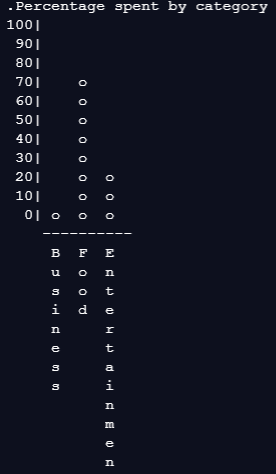# I need help with Budget App: create_spend_chart

Sorry to ask for help on this topic that has already appeared on the forum before. But I am not able to take advantage of other people’s solutions to solve my case.
In principle, the function prints everything fine and 403 characters. But I can’t pass the test, and I’m not able to know why.
Greetings

``````def create_spend_chart(categories):
num_categories = len(categories)
total_expenses = 0
resume_categories = []
num_characters = 0

# collecting data
for category in categories:
expenses = 0
for note in category.ledger:
if note['amount'] < 0:
expenses += abs(note['amount'])
total_expenses += expenses

resume_categories.append({'name': category.name, 'expenses': expenses})
num_characters = max(num_characters, len(category.name))

# printing information
groups = 11  # y axis for scale
letter_index = 0
lines = ['Percentage spent by category']

for line in range(12 + num_characters):
if line < groups:
partial = [f'{100 - line * 10}|'.rjust(pad, " ")]
for category in resume_categories:
category['%expenses'] = round_down(
category['expenses'] / total_expenses * 100, -1)
if (100 - line * 10) <= category['%expenses']:
partial.append('o ')
else:
partial.append('  ')
lines.append(' '.join(partial) + ' ')

if line == groups:
lines.append(" " * pad + "-" * (num_categories * 2 + 4))
if line > groups:
partial = [" " * pad]
for category in resume_categories:
if letter_index < len(category['name']):
partial.append(category['name'][letter_index] + ' ')
else:
partial.append('  ')
lines.append(' '.join(partial) + ' ')
letter_index += 1

print('\n'.join(lines))

def round_down(number, num_decimals=0):
multiplier = 10 ** num_decimals
return math.floor(number * multiplier) / multiplier

Could you show also your implementation of the `Category` class? Having all of the code it will be easier to help.

Without a doubt, thanks for your interest.

``````import math

class Category:
def __init__(self, name):
self.name = name
self.ledger = []

def deposit(self, amount, description=''):
self.ledger.append({'amount': amount, 'description': description})

def withdraw(self, amount, description=''):
if self.check_funds(amount):
self.ledger.append({'amount': -amount, 'description': description})
return True
return False

def get_balance(self):
balance = sum([note['amount'] for note in self.ledger])
return balance

def transfer(self, amount, category):
if self.check_funds(amount):
self.withdraw(amount, f'Transfer to {category.name}')
category.deposit(amount, f'Transfer from {self.name}')
return True
return False

def check_funds(self, amount):
return amount <= self.get_balance()

def __str__(self):
total_characterers = 30
total_amount = 0
pad = total_characterers // 2 - len(self.name) // 2
lines = ''
for note in self.ledger:
total_amount += note['amount']
amount_format = f'{note["amount"]:0.02f}'
lines += f'\n{note["description"][:23].ljust(23)}{amount_format.rjust(7)}'
lines += f'\nTotal: {total_amount:0.02f}'
return lines

def create_spend_chart(categories):
num_categories = len(categories)
total_expenses = 0
resume_categories = []
num_characters = 0

# collecting data
for category in categories:
expenses = 0
for note in category.ledger:
if note['amount'] < 0:
expenses += abs(note['amount'])
total_expenses += expenses

resume_categories.append({'name': category.name, 'expenses': expenses})
num_characters = max(num_characters, len(category.name))

# printing information
groups = 11  # y axis for scale
letter_index = 0
lines = ['Percentage spent by category']

for line in range(groups + 1 + num_characters):
if line < groups:
partial = [f'{100 - line * 10}|'.rjust(pad, " ")]
for category in resume_categories:
category['%expenses'] = round_down(
category['expenses'] / total_expenses * 100, -1)
if (100 - line * 10) <= category['%expenses']:
partial.append('o ')
else:
partial.append('  ')
lines.append(' '.join(partial) + ' ')

if line == groups:
lines.append(" " * pad + "-" * (num_categories * 2 + 4))
if line > groups:
partial = [" " * pad]
for category in resume_categories:
if letter_index < len(category['name']):
partial.append(category['name'][letter_index] + ' ')
else:
partial.append('  ')
lines.append(' '.join(partial) + ' ')
letter_index += 1

print('\n'.join(lines))

def round_down(number, num_decimals=0):
multiplier = 10 ** num_decimals
return math.floor(number * multiplier) / multiplier

``````Function is expected to return the string with the chart, per specification, it doesn’t need nor should print it on it own.

1 Like

Wow, I feel embarrassed.
It has worked as you tell me.
Thank you very much for your help!
I’ve been thinking about it all afternoon.
Thanks!

Failing test was writing that in it own - a bit convoluted way, it can be hard sometimes to figure out what it’s trying to say exactly.

You might have noticed, in the output, somewhere above what is on the image, printed `None`. That was actually what function was returning at the time. When function isn’t returning anything explicitly, python returns `None`.

You are right, I have replicated the problem and see in the error message “AssertionError: None !=…”
Now, I understand the message of the test.

This topic was automatically closed 182 days after the last reply. New replies are no longer allowed.